Courses

# Test: Circles- 1

## 25 Questions MCQ Test UPSC Prelims Paper 2 CSAT - Quant, Verbal & Decision Making | Test: Circles- 1

Description
This mock test of Test: Circles- 1 for Class 9 helps you for every Class 9 entrance exam. This contains 25 Multiple Choice Questions for Class 9 Test: Circles- 1 (mcq) to study with solutions a complete question bank. The solved questions answers in this Test: Circles- 1 quiz give you a good mix of easy questions and tough questions. Class 9 students definitely take this Test: Circles- 1 exercise for a better result in the exam. You can find other Test: Circles- 1 extra questions, long questions & short questions for Class 9 on EduRev as well by searching above.
QUESTION: 1

Solution:
QUESTION: 2

### ABCD is a parallelogram. A circle passes through A and D and cuts AB at E and DC at F. If ∠BEF = 80o, then ∠ABC is equal to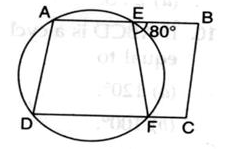Solution:

Given, ABCD is a parallelogram and AEFD is a cyclic quadrilateral.
∠BEF=80
Now, ∠ADC=∠BEF=80 (Angle of a cyclic quadrilateral is equal to the opposite exterior angle )
Also, now in parallelogram ABCD,
∠ABC=∠ADC=80 (Opposite angles of a parallelogram are equal)

QUESTION: 3

### In the given figure, if ∠CDB=40o, then the measure of ∠PAC is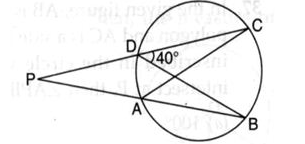Solution:
QUESTION: 4

In the given figure, ABCD is a quadrilateral inscribed in circle with centre O. CD is produced to E. If ∠ADE = 95o and ∠OBA = 30o, then ∠OAC is equal to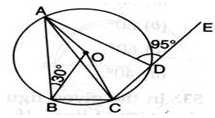Solution: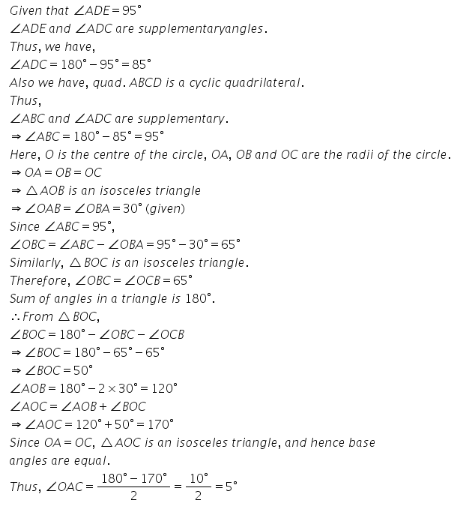QUESTION: 5

In a figure, O is the centre of the circle with AB as diameter. If ∠AOC = 40o, the value of x is equal to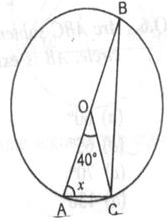Solution:
QUESTION: 6

The given figures show two congruent circles with centre O and O’. Arc AXB subtends an angle of 75o at the centre and arc A’YB’ subtends an angle of 25o at the centre O’. Then, the ratio of arcs AXB to A ‘YB’ is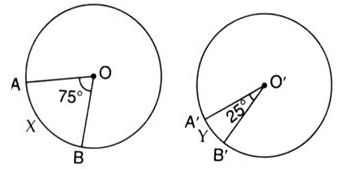Solution:

Because the circles are congruent, therefore their radius are same

Length of arc AXB = [θ/(360°)] × 2πr = [(75°)/(360°)] × 2πr

Length of arc A’YB’ = [θ/(360°)] × 2πr = [(25°)/(360°)] × 2πr

Required Ratio = ([(75°)/(360°)] × 2πr) : ([(25°)/(360°)] × 2πr) = 3 : 1

QUESTION: 7

If a chord of a circle is equal to its radius, then the angle subtended by this chord in major segment is

Solution:
QUESTION: 8

The given figure shows two intersecting circles. If ∠ABC = 75o, then the measure of ∠PAD is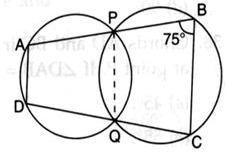Solution:
QUESTION: 9

In the given figure, ABCD is a cyclic quadrilateral in which ∠BAD = 75o, ∠ABD = 58o and ∠ADC = 77o , AC and BD intersect at P. the measure of ∠DPC is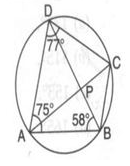Solution:
QUESTION: 10

For what value of x in the figure, points A, B, C and D are concyclic ?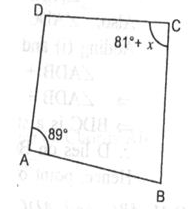Solution:
QUESTION: 11

Greatest chord of a circle is called its

Solution:
QUESTION: 12

In the given figure PQ and RS are two equal chords of a circle with centre O. OA and OB are perpendiculars on chords PQ and RS, respectively. If ∠AOB = 140o, then ∠PAB is equal to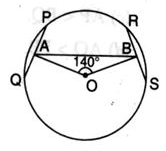Solution:
QUESTION: 13

In the given figure, chords AB and CD intersect at P. If ∠DPB = 88o and ∠DAP = 46o, then the measure of ∠ABC is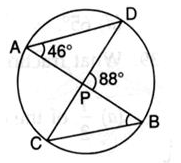Solution:
QUESTION: 14

AD is diameter of a circle and AB is a chord. If AD = 50 cm, AB = 48 cm, then the distance of AB from the centre of the circle is

Solution:
QUESTION: 15

In the given figure, O is the centre of the circle. If ∠CAB = 40o and ∠CBA = 110o, the value of x is :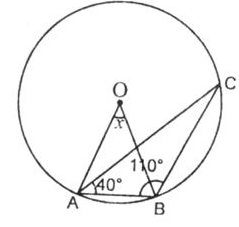Solution:
QUESTION: 16

Angle formed in minor segment of a circle is

Solution:
QUESTION: 17

In the given figure PQ = QR = RS and ∠PTS = 75o then the measure of ∠QOR is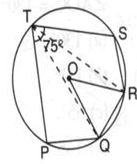Solution:
QUESTION: 18

In the given figure, O is the centre of the circle ABE is a straight line,. If ∠DBE = 95o then ∠AOD is equal to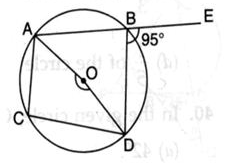Solution:
QUESTION: 19

The given figure shows two congruent circles with centre O and O’ intersecting at A and B. If ∠AO′B = 50o, then the measure of ∠APB is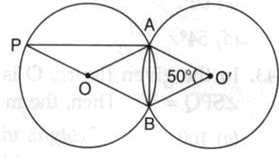Solution:
QUESTION: 20

Angle inscribed in a semicircle is :

Solution:
QUESTION: 21

Number of circles that can be drawn through three non-collinear points is

Solution:
QUESTION: 22

In the given figure, if ∠ABC = 50o and ∠BDC = 40o, then ∠BCA is equal to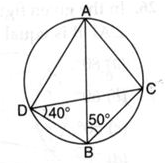Solution:
QUESTION: 23

In the given figure, chords AB and CD intersect each other at right angles. Then, ∠x+∠y is equal to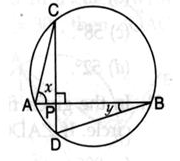Solution:
QUESTION: 24

In the given figure if ∠CAB = 49o and ∠ADC = 43o, then the measure of ∠ACB is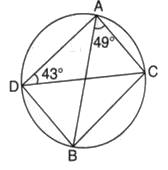Solution:
QUESTION: 25

In the given figure, O is the centre of the circle. If ∠QPR is 50o, then ∠QOR is :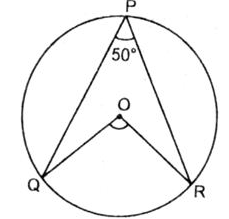Solution: